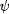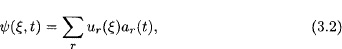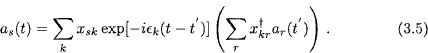# Propagators and Second Quantization

The Schrödinger equation for an electron or for N noninteracting electrons iswith units such that Planck’s constant is 2π and the electron mass is 1, and with ξ = (, ζ) being a combined space-spin variable. The wave function or Schrödinger amplitude(ξ, t) can be expressed in an orthonormal basis {us(ξ)} asgiving the Schrödinger equation in discrete formThe notationhas been employed.

Let x be a unitary transformation to energy eigenstates: xx = xx = 1, xhx = ∊ (diagonal). A formal solution to Eq. (3.3) may be written asThe system may be prepared such that |ar(t′)|2 = 1, and |as(t′)|2 = 0 for sr. Then the quantityis the probability ...

Get Propagators in Quantum Chemistry, 2nd Edition now with the O’Reilly learning platform.

O’Reilly members experience books, live events, courses curated by job role, and more from O’Reilly and nearly 200 top publishers.